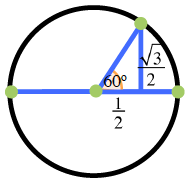### Home > PC > Chapter 4 > Lesson 4.2.1 > Problem4-70

4-70.
1. Given θ =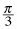, find the exact value of each of the following trig expressions. Homework Help ✎

1. sec θ

2. csc θ

3. tan θ

4. cot θ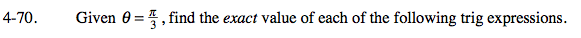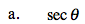$\frac{1}{\text{cos}(\theta)}=\frac{1}{x}=\frac{1}{\frac{1}{2}}=2$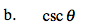What is the cscθ in terms of the other trig functions?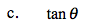What is the tanθ in terms of the other trig functions?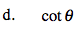What is the cotθ in terms of the other trig functions?

Draw a unit circle and label the x and y-coordinates for θ. You can easily find all trig functions once you know the coordinates.